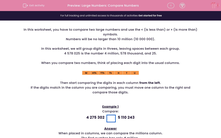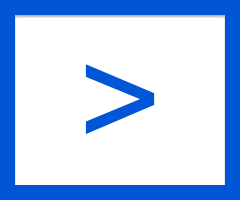# Large Numbers: Compare Numbers

In this worksheet, students compare numbers up to 10 000 000, using the < or > sign between the two given numbers.This content is premium and exclusive to EdPlace subscribers.Key stage:  KS 2

Curriculum topic:   Maths and Numerical Reasoning

Curriculum subtopic:   Place Value

Difficulty level:#### Worksheet Overview

In this worksheet, you have to compare two large numbers and use the < (is less than) or > (is more than) symbols.

Numbers will be no larger than 10 million (10 000 000).

In this worksheet, we will group digits in threes, leaving spaces between each group.

4 578 025 is the number 4 million, 578 thousand, and 25.

When you compare two numbers, think of placing each digit into the usual columns.Then start comparing the digits in each column from the left.

If the digits match in the column you are comparing, you must move one column to the right and compare those digits.

Example 1

Compare:

4 275 3025 110 243

When placed in columns, we can compare the millions column.

The first number has only 4 million.

The second number has 5 million.

4 275 3025 110 243

Example 2

Compare:

4 275 3024 210 243

When placed in columns, we can compare the millions column.

They are both 4 million.

So we go to the next column – the hundred thousands column.

They are both 2 hundred thousand.

So we go to the next column – the ten thousands column.

The first number has 7 in this column.

The second number has 1 in this column.

4 275 3024 210 243

Example 3

Compare:

275 3022 710 243

When placed in columns, the number on the right has the digit 2 in the millions column.

The number on the left doesn't have a digit in the millions column. Call it zero.

0 < 2

275 3022 710 243

### What is EdPlace?

We're your National Curriculum aligned online education content provider helping each child succeed in English, maths and science from year 1 to GCSE. With an EdPlace account you’ll be able to track and measure progress, helping each child achieve their best. We build confidence and attainment by personalising each child’s learning at a level that suits them.

Get started